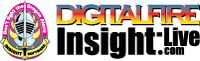•The secret to cool bodies and glazes is a lot of testing.
•The secret to know what to test is material and chemistry knowledge.
•The secret to learning from testing is documentation.
•The place to test, do the chemistry and document is an account at https://insight-live.com
•The place to get the knowledge is https://digitalfire.com

Sign-up at https://insight-live.com today.

# FX Ball Clay

 Oxide Analysis Formula CaO 0.30% 0.020 MgO 1.01% 0.094 K2O 2.62% 0.104 Na2O 1.01% 0.061 TiO2 1.51% 0.071 Al2O3 27.22% 1.000 SiO2 57.67% 3.596 Fe2O3 0.90% 0.021 LOI 7.76 Oxide Weight 345.65 Formula Weight 374.72

## XML for Import into INSIGHT

<?xml version="1.0" encoding="UTF-8"?> <material name="FX Ball Clay" descrip="" searchkey="" loi="7.76" casnumber="70694-09-6"> <oxides> <oxide symbol="CaO" name="Calcium Oxide, Calcia" status="" percent="0.300" tolerance=""/> <oxide symbol="MgO" name="Magnesium Oxide, Magnesia" status="" percent="1.010" tolerance=""/> <oxide symbol="K2O" name="Potassium Oxide" status="" percent="2.620" tolerance=""/> <oxide symbol="Na2O" name="Sodium Oxide, Soda" status="" percent="1.010" tolerance=""/> <oxide symbol="TiO2" name="Titanium Dioxide, Titania" status="" percent="1.510" tolerance=""/> <oxide symbol="Al2O3" name="Aluminum Oxide, Alumina" status="U" percent="27.220" tolerance=""/> <oxide symbol="SiO2" name="Silicon Dioxide, Silica" status="" percent="57.670" tolerance=""/> <oxide symbol="Fe2O3" name="Iron Oxide, Ferric Oxide" status="" percent="0.900" tolerance=""/> </oxides> <volatiles> <volatile symbol="LOI" name="Loss on Ignition" percent="7.760" tolerance=""/> </volatiles> </material>